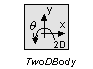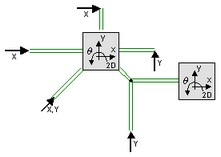﻿ 20-sim webhelp > Library > Iconic Diagrams > Mechanical > Translation > 2DSmallRotation > TwoDBody

# TwoDBody## Library

Iconic Diagrams\Mechanical\Translation\2DSmallAngles

## Use

Domains: Continuous. Size: 2-D. Kind: Iconic Diagrams (2D Planar).

## Description

The central model in the 2DSmallRotation library is the TwoDBody model. It describes a body with three degrees of freedom: x, y and q. The TwoDBody is a point mass with rotational inertia. The constitutive equations are therefore very simple:

P.v = I .* int(P.F);

With

I = [1/M; 1/M; 1/J];

Any number of connections can be made to the TwoDBody model. All forces acting upon the body are added.Because any number of connections can be made, successive ports are named P1, P2, P3 etc. 20-sim will automatically create equations such that the resulting force P.F is equal to the sum of the forces of all connected ports P1 . Pn. The velocities of all connected ports are equal to P.v. The model has a preferred velocity out causality. The corresponding constitutive equations then contain an integration. The model can also have the non-preferred force out causality. The constitutive equations then contain a differentiation.

P.F = sum(P1.F, P2.F, ....)

P.v = P1.v = P2.v = ....

velocity out causality (preferred):

I = [1/M;1/M;1/J]

P.v = I*int(P.F);

force out causality:

I = [1/M;1/M;1/J]

P.F = I-1*ddt(P.v);

## Interface

 Ports Description P Port with three degrees of freedom (x, y, q). Any number of connections can be made. Causality preferred velocity out Parameters M J Mass [kg]. Moment of inertia [kgm2/rad]

## Note

 • A TwoDBody model can be connected to any other element of the 2D-library except other 2D-bodies. Two bodies can always be simplified to one TwoDBody model with the combined mass and inertia of both single bodies.
 • TwoDBody models can be connected to 1D models using the TwoDPoint models as intermediate. If a TwoDPoint is connected to a body, it constrains the movement of the body in one direct